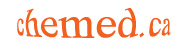The last exam question

I've been told that this question is 'impossible.' Hmm...

 13. A chemist in a forensic laboratory is asked to identify the substance responsible for the death of a controversial politician, who appears to have been poisoned. He isolates the substance and is able to obtain the following information about it:

a) The substance is insoluble in pure water, but dissolves in acid, forming a gas. The gas does not support combustion in air. When the gas is passed into water, a slightly acidic solution results. The density of the gas at 298 K and 1.000 atm pressure is 1.7998 g/L.

b) When a solid sample of the original poison substance is heated, it decomposes, releasing a gas. The resulting solid dissolves in water to give a basic solution.

c) When the dry solid from (b) above is tested, it is found to have a molecular mass of 424.766 g/mol. Also, it is found to react with two moles of sodium to give a shiny, hard substance in addition to a compound of sodium

Explain the significance of each of the observations above in a sentence or two, identify the gas in a) and the solid in b) and identify the original poison substance.

About a quarter of the students writing this exam got it - full marks, or close to it. For the most part, students who did well on this question did very well on the rest of the exam, indicating that the question discriminated effectively among the better students. In other words, a fair but hard question.

There are several ways to get the 'right' answer. The question contains more information than is actually required to find the solution. There were multiple 'wrong' answers that got almost marks.

The solution

Here we go...

 13. A chemist in a forensic laboratory is asked to identify the substance responsible for the death of a controversial politician, who appears to have been poisoned. He isolates the substance and is able to obtain the following information about it:

No, it's not dioxin. But the question may have been inspired by the botched Ukrainian election. Note that Yushchenko was not killed, just disfigured.

a) The substance is insoluble in pure water, but dissolves in acid, forming a gas. The gas does not support combustion in air. When the gas is passed into water, a slightly acidic solution results. The density of the gas at 298 K and 1.000 atm pressure is 1.7998 g/L.

Use the data to calculate the molecular mass: M = dRT/P. Molecular mass = dirt / pressure... where d = density. The rest is obvious.

Now we have a gas of molecular mass 44 g/mol which is formed when the substance is dissolved in acid. Sure sounds like CO2. It helps that many carbonates are insoluble. Nitrous oxide (dinitrogen oxide) has a similar molecular weight, but it wouldn't form this way.

b) When a solid sample of the original poison substance is heated, it decomposes, releasing a gas. The resulting solid dissolves in water to give a basic solution.

Heating a metal carbonate can form a metal oxide + a carbon dioxide. Metal oxides, being ionic, contain the oxide ion, which is basic.

c) When the dry solid from (b) above is tested, it is found to have a molecular mass of 424.766 g/mol. Also, it is found to react with two moles of sodium to give a shiny, hard substance in addition to a compound of sodium.

The solid from b is the metal oxide. Subtract the mass of a mole of oxygen atoms from 424.766 g/mol to get the mass of the metal - which is greater than that of any known element. But it reacts with two moles of Na in an obvious redox reaction (the shiny hard substance is a metal), so there must be two electrons per mole being transferred, meaning that there must be two atoms of metal in the formula. A bit of arithmetic leads to thallium - which is most stable in the +1 state, thanks to the relativistic inert pair effect.

My thoughts

God, I'm such a bastard. Imagine asking University students to think!!